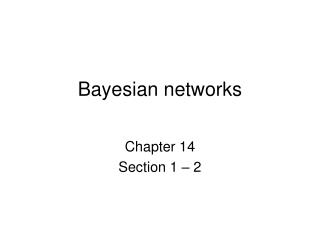DownloadDownload PresentationBayesian networks

# Bayesian networks

Télécharger la présentation## Bayesian networks

- - - - - - - - - - - - - - - - - - - - - - - - - - - E N D - - - - - - - - - - - - - - - - - - - - - - - - - - -
##### Presentation Transcript

1. Bayesian networks Chapter 14 Section 1 – 2

2. Outline • Syntax • Semantics

3. Bayesian networks • A simple, graphical notation for conditional independence assertions and hence for compact specification of full joint distributions • Syntax: • a set of nodes, one per variable • a directed, acyclic graph (link ≈ "directly influences") • a conditional distribution for each node given its parents: P (Xi | Parents (Xi)) • In the simplest case, conditional distribution represented as a conditional probability table (CPT) giving the distribution over Xi for each combination of parent values

4. Example • Topology of network encodes conditional independence assertions: • Weather is independent of the other variables • Toothache and Catch are conditionally independent given Cavity

5. Example • I'm at work, neighbor John calls to say my alarm is ringing, but neighbor Mary doesn't call. Sometimes it's set off by minor earthquakes. Is there a burglar? • Variables: Burglary, Earthquake, Alarm, JohnCalls, MaryCalls • Network topology reflects "causal" knowledge: • A burglar can set the alarm off • An earthquake can set the alarm off • The alarm can cause Mary to call • The alarm can cause John to call

6. Example contd.

7. Compactness • A CPT for Boolean Xi with k Boolean parents has 2k rows for the combinations of parent values • Each row requires one number p for Xi = true(the number for Xi = false is just 1-p) • If each variable has no more than k parents, the complete network requires O(n · 2k) numbers • I.e., grows linearly with n, vs. O(2n)for the full joint distribution • For burglary net, 1 + 1 + 4 + 2 + 2 = 10 numbers (vs. 25-1 = 31)

8. Semantics The full joint distribution is defined as the product of the local conditional distributions: P (X1, … ,Xn) = πi = 1P (Xi | Parents(Xi)) e.g., P(j  m  a b e) = P (j | a) P (m | a) P (a | b, e) P (b) P (e) n

9. Constructing Bayesian networks • 1. Choose an ordering of variables X1, … ,Xn • 2. For i = 1 to n • add Xi to the network • select parents from X1, … ,Xi-1 such that P (Xi | Parents(Xi)) = P (Xi | X1, ... Xi-1) This choice of parents guarantees: P (X1, … ,Xn) = πi =1P (Xi | X1, … , Xi-1) (chain rule) = πi =1P (Xi | Parents(Xi)) (by construction) n n

10. Example • Suppose we choose the ordering M, J, A, B, E P(J | M) = P(J)?

11. Example • Suppose we choose the ordering M, J, A, B, E P(J | M) = P(J)? No P(A | J, M) = P(A | J)?P(A | J, M) = P(A)?

12. Example • Suppose we choose the ordering M, J, A, B, E P(J | M) = P(J)? No P(A | J, M) = P(A | J)?P(A | J, M) = P(A)? No P(B | A, J, M) = P(B | A)? P(B | A, J, M) = P(B)?

13. Example • Suppose we choose the ordering M, J, A, B, E P(J | M) = P(J)? No P(A | J, M) = P(A | J)?P(A | J, M) = P(A)? No P(B | A, J, M) = P(B | A)? Yes P(B | A, J, M) = P(B)? No P(E | B, A ,J, M) = P(E | A)? P(E | B, A, J, M) = P(E | A, B)?

14. Example • Suppose we choose the ordering M, J, A, B, E P(J | M) = P(J)? No P(A | J, M) = P(A | J)?P(A | J, M) = P(A)? No P(B | A, J, M) = P(B | A)? Yes P(B | A, J, M) = P(B)? No P(E | B, A ,J, M) = P(E | A)? No P(E | B, A, J, M) = P(E | A, B)? Yes

15. Example contd. • Deciding conditional independence is hard in noncausal directions • (Causal models and conditional independence seem hardwired for humans!) • Network is less compact: 1 + 2 + 4 + 2 + 4 = 13 numbers needed

16. Summary • Bayesian networks provide a natural representation for (causally induced) conditional independence • Topology + CPTs = compact representation of joint distribution • Generally easy for domain experts to construct